##### Trigonometry For Dummies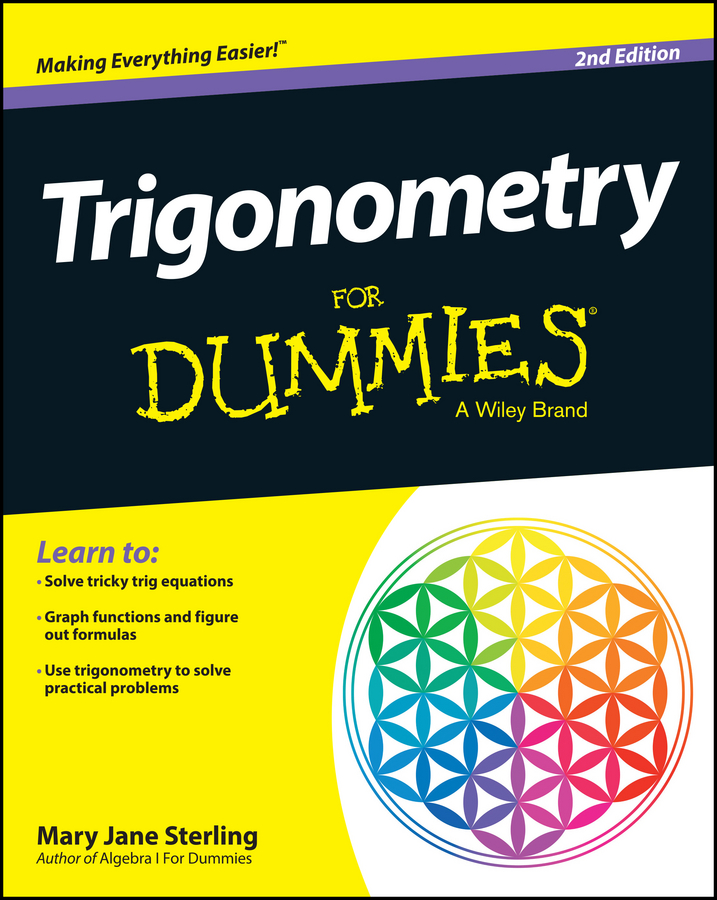When you have two angles in a triangle and the side between them (ASA), you can use trig to find the area of the triangle. The formulas go as follows.

In triangle ABC, if the measures of the sides are a, b, and c opposite the respective angles, then you can determine the area by using one of the following equations: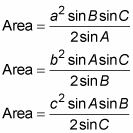These formulas are actually built from the formula for finding the area with SAS (side-angle-side), with a little help from the law of sines. Here’s how one of them came to be.

1. Start with the SAS rule for area.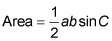2. Write the law of sines involving angles A and B.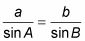3. Solve for b.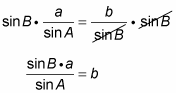4. Substitute the equivalent for b into the area formula.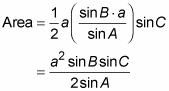Now consider an example. Say you have a triangle with angles A, which is 45 degrees, and B, which is 55 degrees, and the side between them, c, equal to 10. Find the area.

1. Choose the correct formula — the one with c2 in it.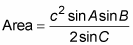2. Find the sines of the two given angles.

The sine of 45 degrees equals 0.707, and the sine of 55 degrees equals 0.819.

3. Find the sine of the third angle.

Angle C measures 180 – (45 + 55), or 180 – 100, which equals 80 degrees.

The sine of 80 degrees equals 0.985.

4. Substitute the values into the formula and solve.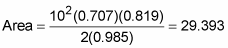The area is a little over 29 square units.